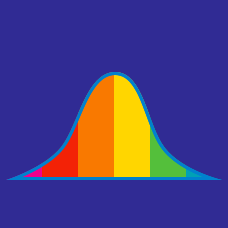Probability

# Understanding Data: Level 2 Challenges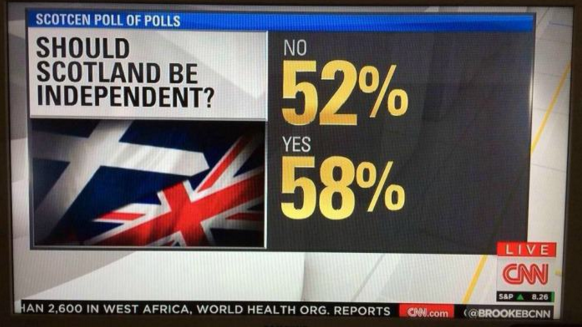What is wrong with this news coverage?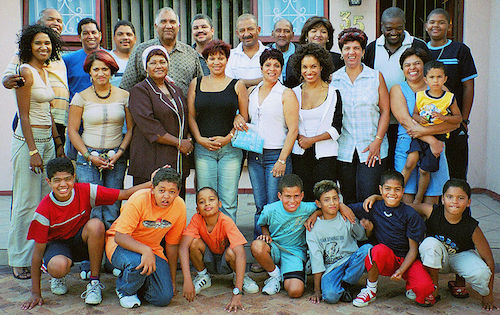The average age of the family (father, mother, and several children) is 18. If the father, who is 38 years old, is not included, then the (new) average would be 14. Find the number of children in the family.

There are eight people in a family. One of the members receives $x$ presents. Five members receive $x+4$ each and the rest only get $x-2$ each.

Consider the 8 numbers of presents received, and find $2 \times \mathrm{mode} - \mathrm{mean} - \mathrm{median}.$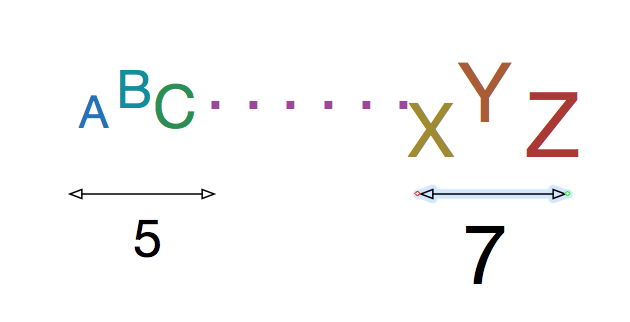The sum of $N$ real numbers (not necessarily unique) is 20. The sum of the 3 smallest of these numbers is 5. The sum of the 3 largest is 7. Which of the following are possible values for $N$?

I. 9
II. 10
III. 11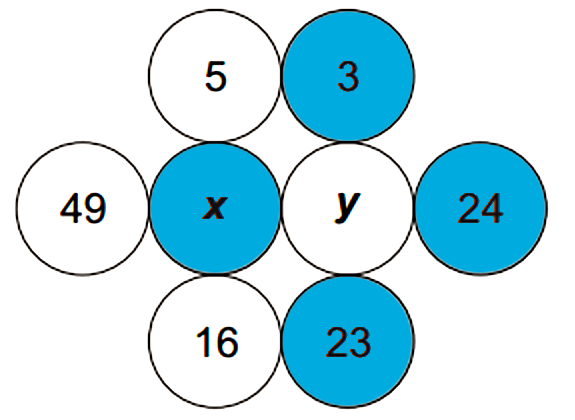The value of $x$ is the mean of the numbers in the white circles. The values of $y$ is the mean of numbers in the blue circles.

Find $x-y$.

×